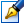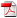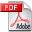##Models of discrete optimal control problem and solving dynamic games on networks

 Author: Anatoli Iusiumbeli Degree: doctor of physics and mathematics Speciality: 01.01.09 - Mathematical cybernetics and operation research Year: 2007 Scientific adviser: Dumitru Lozovanudoctor habilitat, professor, Moldova State University Institution: Scientific council:

### Status

The thesis was presented on the 24 August, 2007
Approved by NCAA on the 18 October, 2007

### Abstract0.44 Mb / in romanian

### Thesis

CZU 519.61.60 Mb / in romanian
82 pages

### Keywords

optimal control problem, optimal solution in sense of Nash, regular condition, c-games, dynamic programming

### Summary

In the dissertation the problem of discrete control on the dynamic networks is under research. The basic methods for studying and solving these problems are based on the principle of dynamic programming. The method of dynamic programming for optimal control problems is extended when the dynamic of the system is described by an oriented weighted graph in which vertices correspond with the states of the system and its edges show the possibility for the system to pass from one state to another one in every moment of time. Some results which argue and prove the adequacy of the elaborated algorithms for solving the optimal control problem on networks are given. The generalization of the Dijkstra’s algorithm for finding the optimal paths if the dynamic system satisfy the regular condition is obtained. The modification of the algorithm of finding the tree of the minimum paths on the dynamic networks is proposed.

The game variant of the optimal discrete control problem on the dynamic networks with integral cost function in the case when the initial and final states are fixed is under research, too. In the dissertation it is shown that researching and solving these problems can be reduced to determining of the optimal strategies in so-called c-games. The dynamic c-games are studied. They are connected with the multicriterion optimal control problem in its positional form. The fact that the game variant of the optimal control problem can be reduced to a special class of c-games on networks is proved.

The problem of finding the point of equilibrium in sense of Nash for the multicriterion optimal control problem is researched. The solution of the considered problem is the solution of a dynamic noncooperative game. The necessary and sufficient conditions of existence of the point of the equilibrium in sense of Nash are found. Polynomial-time algorithms for finding the optimal strategies of the players based on the obtained results are elaborated.

The case with a fixed number of passages and the case with an unlimited number of passages are considered separately.

The proposed algorithms have been tested using concrete examples. Program on C++ for some of the algorithms is elaborated